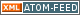www.LinuxHowtos.org howtos, tips&tricks and tutorials for linuxfrom small one page howto to huge articles all in one place

search text in:

Poll
Which filesystem do you use?

poll results

using iotop to find disk usage hogs

using iotop to find disk usage hogs

words:

887

views:

132588

userrating:

average rating: 1.7 (87 votes) (1=very good 6=terrible)

May 25th. 2007:
Words

486

Views

239067

Workaround and fixes for the current Core Dump Handling vulnerability affected kernels

Workaround and fixes for the current Core Dump Handling vulnerability affected kernels

words:

161

views:

119982

userrating:

average rating: 1.3 (28 votes) (1=very good 6=terrible)

April, 26th. 2006:

You are here: manpages

COMPLEX

Section: Linux Programmer's Manual (7)
Updated: 2011-09-16

NAME

complex - basics of complex mathematics

SYNOPSIS

#include <complex.h>

DESCRIPTION

Complex numbers are numbers of the form z = a+b*i, where a and b are real numbers and i = sqrt(-1), so that i*i = -1.

There are other ways to represent that number. The pair (a,b) of real numbers may be viewed as a point in the plane, given by X- and Y-coordinates. This same point may also be described by giving the pair of real numbers (r,phi), where r is the distance to the origin O, and phi the angle between the X-axis and the line Oz. Now z = r*exp(i*phi) = r*(cos(phi)+i*sin(phi)).

The basic operations are defined on z = a+b*i and w = c+d*i as:

addition: z+w = (a+c) + (b+d)*i
multiplication: z*w = (a*c - b*d) + (a*d + b*c)*i
division: z/w = ((a*c + b*d)/(c*c + d*d)) + ((b*c - a*d)/(c*c + d*d))*i

Nearly all math function have a complex counterpart but there are some complex-only functions.

EXAMPLE

Your C-compiler can work with complex numbers if it supports the C99 standard. Link with -lm. The imaginary unit is represented by I.

/* check that exp(i * pi) == -1 */ #include <math.h> /* for atan */ #include <stdio.h> #include <complex.h>

int main(void) {
double pi = 4 * atan(1.0);
double complex z = cexp(I * pi);
printf("%f + %f * i\n", creal(z), cimag(z)); }

cabs(3), cacos(3), cacosh(3), carg(3), casin(3), casinh(3), catan(3), catanh(3), ccos(3), ccosh(3), cerf(3), cexp(3), cexp2(3), cimag(3), clog(3), clog10(3), clog2(3), conj(3), cpow(3), cproj(3), creal(3), csin(3), csinh(3), csqrt(3), ctan(3), ctanh(3)

COLOPHON

This page is part of release 4.13 of the Linux man-pages project. A description of the project, information about reporting bugs, and the latest version of this page, can be found at https://www.kernel.org/doc/man-pages/.

Index

NAME
SYNOPSIS
DESCRIPTION
EXAMPLE
COLOPHON

Other free services
toURL.org
Shorten long
URLs to short
http://tourl.org/2
tourl.org
.
Reverse DNS lookup
Find out which hostname(s)
resolve to a
given IP or other hostnames for the server
www.reversednslookup.org||- Powered by- Running on-
Copyright 2004-2018 Sascha Nitsch Unternehmensberatung UG(haftungsbeschränkt)::- Level Triple-A Conformance to Web Content Accessibility Guidelines 1.0 -
- Copyright and legal notices -
Time to create this page: 3.8 ms